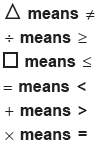# User ForumSubject :IMO    Class : Class 6

In the given question,If a + b and b + c then_____.

(A) c ÷ a

(B) a = c

(C) a⁎ c

(D) c = a
how to get the answer

## Ans 1:

Class : Class 9
Plus sign means is smaller than so a is smaller than b ; b is smaller than c. That means c is smaller than a. = means is smaller than so c = a. answer is D

## Ans 2:

Class : Class 7
Answer is option D